International Journal of Science, Technology and Society
Volume 3, Issue 4, July 2015, Pages: 214-219

An Overview on Voltage Stability Indices as Indicators of Voltage Stability for Networks with Distributed Generations Penetration

Haruna Musa

Department of Electrical Engineering, Bayero University, Kano, Nigeria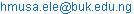Haruna Musa. An Overview on Voltage Stability Indices as Indicators of Voltage Stability for Networks with Distributed Generations Penetration. International Journal of Science, Technology and Society. Vol. 3, No. 4, 2015, pp. 214-219. doi: 10.11648/j.ijsts.20150304.26

Abstract: The increase in Distributed Generation (DG) penetration in distribution network can be used as a means of addressing the increasing load demand without the upgrade of transmission lines. However, this increasing demand despite the supplementary supply from DG sources can cause the system to operate at its maximum capacity or at point of voltage collapse. It therefore, becomes necessary to determine the maximum capacity limit of the system before voltage collapse occurs due to instability. This paper reviews the concept of voltage stability index (VSI) as an indicator of a weak bus that is closed to its maximum allowable limit or the  most critical line to voltage collapse in a network. The review also evaluates various voltage stability indices including those originally developed for transmission systems. Subsequently, their adequacies are quantitatively compared and from the comparison, it is observed that the existing voltage stability indices would be inadequate for assessing the most sensitive bus or line on the verge of voltage collapse for modern distribution systems.

Keywords: Distributed Generation, Penetration, Voltage Stability, Voltage Stability Index, Voltage Collapse

1. Introduction

The impact of DG on distribution network is positive as it improves voltage stability of a network apart from other improvement, such as power loss reduction, voltage profile improvement and power quality improvement provided it is well sited. The improvement in the voltage profiles at load terminals vary depending on their relative locations with respect to the switching point. In general, analysis of voltage performance shows that DG can support and improve the voltage profiles at load terminals. This can extend the stability margin of dynamic loads, which can result in loss of stability with voltage dips. One of the main factors restricting increase of load served by distribution system is the voltage stability which many distribution companies consider as one of the planning objectives especially with increased penetration of DG in the existing networks . The increase is due to the fact that DG has brought new changes to traditional power system and their connection to distribution networks has improved the voltage stability of the network .

In voltage stability assessment one of the most important considerations is to know the distance to maximium loadability point from the current operating point. One of the methods for such assessment is by use of Voltage Stability Index (VSI) which many researchers have attempted using in order to find the distance between initial loading points to the maximum loadbility point [3-6]. This index gives a measure of how far the present operating point is to voltage collapse point which is expressed in term of real or/and reactive power as well as the line parameters.

A number of methods for voltage stability analysis have been tried by many researchers such as P-V and Q-V analysis, Modal Analysis, voltage instability proximity indicator, multiple load flow solutions based indices, Line stability index, Line stability Factor, Reduced Jacobian Determinant, Minimum Singular Value of Power Flow Jacobian, and other voltage indices methods . All these methods are used for the determination of the distance to maximium loadability point from the current operating point.

2. Voltage Stability

Voltage stability is defined as the ability of a system to maintain voltage at all nodes within the acceptable limits when subjected to disturbance . Normally a voltage stable power system is capable of maintaining the post-fault voltages near the pre-fault value. However, if the system is unable to maintain the voltage within acceptable limits, the system can suffer from voltage collapse . In general for the purpose of simulation these methods are categorized into two categories namely the static and dynamic. The dynamic voltage instability is the major cause of interruptions due to the non-linear nature of load involved unlike the static voltage instability that uses linear load and employs assumption that the system is operating in steady state .

2.1. Static Voltage Stability with DG

Static voltage stability analysis can be conducted by assuming the system is operating in the steady state and the load is a linear load. The static load models are expressed interms of active and reactive powers which are functions of the bus voltages.

The level of penetration and the type of DG always determine the nature of impact it will have on the power system. At the same time these two parameters always determines the voltage collapse limit. Networks with high induction generation DG penetration have the tendency of weakening the system voltage stability much more than DG with high penetration of synchronous generation. The reason is that induction machines cannot generate and control reactive power instead they consume reactive power from the system. Also the number of central generations with voltage control ability is lower when part of the demand is supplied by DG units and this can results in less reactive power supply from the central generation .

For the purpose of supplying extra power needed by the load during heavy load demand which if not supplied can results in instability that can lead to voltage collapse, DGs can be embedded in the distribution system to supply the extra load demand as proposed in  for the purpose of maintaining system stability.

2.2. Dynamic Voltage Stability with DG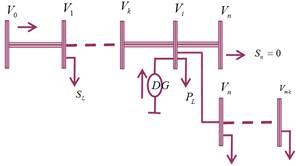Fig 1.0. Radial Distribution feeder with DG.

The rapid increase of generators especially wind turbine in to the power system can have big influence on the power quality and voltage stability of entire network. The optimal placement and sizing of DG in radial distribution system as proposed for capacitor is employed with n bus, laterals and sub-laterals as shown in Fig 1.0 for radial distribution system .

The intermittent nature of wind and the characteristic of wind generators can cause serious problem to the power system as well . Voltage instability problem can be more prominent if induction motors are the main load, since a decrease in its terminal voltage will increase the reactive power consumption greatly even though the active power consumption will slightly decrease. For the purpose of avoiding unintentional islanding during fault condition, the wind turbines are immediately disconnected at the same time other sources are made to increase generation so as to cover for the load around these turbines.

In situations where large numbers of turbines are connected with grid during short-term fault, voltage stability problem can occur due to high reactive power consumption of the generators. This is one of the main technical challenges with large wind generation penetration which usually leads to transient instability that can results in switch off of a large number of the wind generators.

The induction generators are the most popular generators for wind turbines and these generators absorbs reactive power during normal operation which can create low voltage issue in the power system. Furthermore the power flow pattern and system dynamics characteristics changes with high penetration of wind generators into the grid. This therefore calls for system dynamic simulations to ensure the stability of the power system.

3. Evaluation of Voltage Stability by Using Voltage Stability Indices (VSI)

Even though voltage stability is a dynamic problem, static indexes still plays a very important role in voltage stability analysis and helps operators to know how close the current operation point is to static stability limit. Voltage stability index (VSI) was introduced as far back as early 80`s together with other computational methods for evaluating voltage stability in networks which has resulted in opening of new perspective for predicting voltage collapse . The VSI formula is derived from simple current flow equation based on figure 2.0 that indicates the sending and receiving buses as 1 and 2 respectively.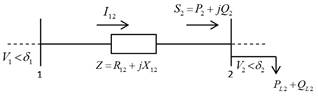Fig. 2.0. Simple 2 bus system for a network.

The VSI has been identified as one of the useful index that could facilitate early prediction of instability in any network. Despite the useful perfections of the existing method, urgent attention is still required in the area for the introduction of newer formulations in order to fully exploit the advantages offered by these methods. The advances in formulations are actually needed for enhancement of capability for detecting instability and early warning signal to system operators. Many formulations were derived to represent the VSI as an indicator of voltage collapse. Among the most popular equations is Fast Voltage Stability Index (FVSI) which was proposed by . In their equation, the authors assumed the power angle between the buses in the network to be small and hence equated to zero. Thus, the formula for FVSI becomes simpler and easier to analyze.

In addition to that, the analysis on VSI was also employed in other power system analysis by using VSI as a constraint as proposed by  where the voltage stability indices were included in the optimal power flow algorithm as a constraint during the load curtailment procedure. By implementing this idea, the total capacity of load curtailment was found to increase as voltage stability margin increases for the system. However, the proposed algorithm is restricted to large system with large loads if better solutions are required. Besides that,  have used the VSI value as an indicator during optimization of reactive power in a network. In this study, the reactive power was found to be an important factor in the network stabilization by means of VSI indicator. The reactive power injection was minimized during the optimization. In another development, the concept of lower voltage limit in the analysis of VSI was introduced by  in order to improve the analysis of VSI to become much faster than traditional VSI. The authors used the angle parameter as a VSI indicator to determine how far the system is from the collapse point.

Moreover, the analysis on VSI is not only to the transmission or to the grid network as been discussed earlier but was also extended to distribution network. Use of VSI was proposed by [19-20], on distribution network and the only difference with analyses on transmission network is on those line parameters that cannot be simplified due to big difference in their values as load changes. For transmission analysis, the authors assumed that the difference in power angle between two buses is small and as such the assumption cannot be applied to distribution systems. In some cases some researchers have assumed that the sending voltage is very close to receiving voltage. All these assumptions or simplification cannot be used for the analysis due to the high R/X ratio associated with the of distribution systems. Furthermore, with the existence of DG in the distribution network the VSI value will differ from what it used to be.

The static indexes that are currently used by system planners and operators are four and these indexes are all kept less than the critical value for system stable operation. All the four indexes are based on power flow concept [21-22]. The four indexes are fast voltage stability index (FVSI), line stability index (LMM), on line stability index (LVSI) and line stability factor (LQP).

The voltage stability index can be evaluated at all nodes for radial distribution as presented by . The equations for the indices were formulated for solution in load flow problem for radial distribution system.

3.1. Fast Voltage Stability Index (FVSI)

This VSI was proposed by  based on simple two bus system having sending and receiving bus. The sending bus is assumed to be the reference bus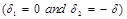and employing this assumption to the index in equation (1) simplifies the expression;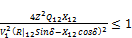(1)

The fast voltage stability index (FVSI) is the simplified equation is expressed as;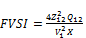(2)

From this expression, the FVSI is directly proportional to reactive power while active power is indirectly related through the bus voltage.

3.2. Line Stability Index (Lmn)

This index was formulated by  and was also based on power flow through a transmission line using п model representation for a two bus system. The power at the receiving end is expressed as;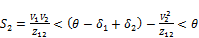(3)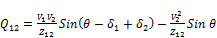(4)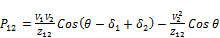(5)

where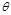is the angle of the line impedance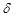is the angle difference between the supply voltage and the receiving end voltage.

Putting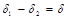into (4) and solving for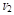;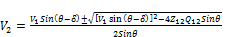(6)

For real value of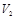, the discriminate of equation (6) must be greater than or equal to zero. This is a stability criteria which must be satisfied as;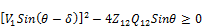(7)

Since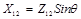the line index is expressed as;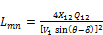(8)

The line index is also directly related to the reactive power and indirectly related to the active power through the voltage.

3.3. Online Stability Index (LVSI)

The online stability index expresses the relationship between line active power and the bus voltage of a line as proposed by [26-27]. By using the discriminant of voltage quadratic equation which must be greater or equal to zero, the receiving end bus voltagecan be derived from equation (5) as;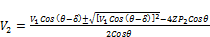(9)

The discriminant of equation (9) is;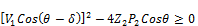(10)

For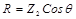(11)

Therefore, LSVI is defined as;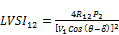(12)

The LVSI relationship is a direct relationship with active power of the receiving end bus and is indirectly related with reactive power through the sending end voltage unlike the case for FVSI and Lmn.

3.4. Line Stability Factor (LQP)

Using the same concept of load flow studies  has derived the LQP relationship such that the active and reactive power on receiving bus can be expressed as;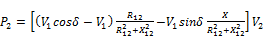(13)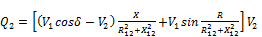(14)

The assumption adopted is that the line is a lossless line with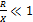and equations (13) and (14) can then be expressed as;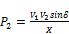(15)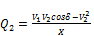(16

Then employing the trigonometry identity giving by;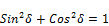(17)

Equations (15) and (16) can be expressed as;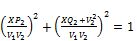(18)

Thus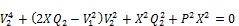(19)

Equation (18) is quadratic equation and for real solution the discriminant must be greater or equal to zero.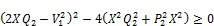(20)

For a lossless line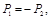then;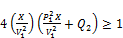(21)

The LQP is expressed as;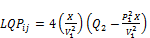(22)

The relationship here is that both active and reactive powers are directly related.

4. Comparison of Indexes

The comparison of the four indexes which are all based on a line connecting between two buses is to establish their critical values based on variables involved and the necessary assumption to be made (if any) as shown in Table 1. Evaluation of these figures will determine the closeness of the line to its transmission limit and at this point any small increase in load can lead to voltage collapse of the entire system for a static load model.

Table 1. Comparison of the Indexes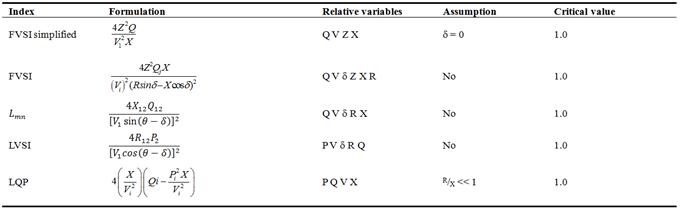For the simplified FVSI where the formulation is based on the assumption that the voltage angle difference is zero, the implication is that such assumption is only applicable to lightly loaded lines and cannot be applicable to heavily loaded lines since the difference is large. The case of FVSI and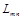is however different in the sense that the two are equivalent and are having direct relations with the receiving end reactive power and indirect relationship with the bus voltage of the sending bus. They are therefore more sensitive to reactive power changes. In the case of LVSI the relationship is direct with active power on receiving end while the bus voltage on sending bus LVSI is more sensitive to d than FVSI and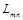because Cos (q - d) changes much faster than (q - d) by around 900 and when q - d approaches 900, Cos (q - d) approaches zero as Cos (q - d) is in the denominator. There may be a dramatic increase in LVSI due to this influence. It implies that a healthy line may be identified as a critical line by LVSI (bottleneck). From all the indexes only LQP have direct relationship with both active and reactive power on the receiving end. Hence, LQP may perform well for either reactive power change or active power change, and is likely going to be better than FVSI and.

5. Conclusions

An overview on voltage stability indices for DG penetrated networks was presented in this paper. The penetration has great improvement on power quality supplied to consumers but has impact on voltage stability of the entire network. This paper consequently, reviews the techniques for the determination of maximum capacity limit for a system before voltage collapse occurs using voltage stability index. The paper also discusses the adequacies of the indices for assessing the most sensitive bus or line on the verge of voltage collapse for modern distribution systems. Out of the indices the line stability factor (LQP) is the only index that directly relates active and reactive power of the receiving end bus and is the most likely index that can perform better for modern distribution systems.

Acknowledgements

The author H. Musa acknowledges with gratitude the financial support offered by Bayero University Kano Nigeria and the provision of suitable research facilities.

References

1. Prada, R.B.; Souza, L.J.; "Voltage stability and thermal limit: constraints on the maximum loading of electrical energy distribution feeders", IEE Proceedings-Generation, Transmission and Distribution, Volume 145, Issue 5, Sept. 1998 Page(s) : 573 – 577
2. N. G. A. Hemdan and M. Kurrat, "Efficient integration of distributed generation for meeting the increased load demand," Int. J. Electr. Power Energy Syst., vol. 33, no. 9, pp. 1572–1583, Nov. 2011.
3. D. Devaraj and J.P. Roselyn, On-line voltage stability assessment using radial basis function network model with reduced input features, International Journal of Electrical Power & Energy Systems, vol. 33, no. 9, Nov 2011, pp. 1550-1555.
4. M.S. Kumar and P. Renuga, Application of Bacterial Foraging Algorithm for Optimal Location of FACTS Devices with Multi-Objective Functions, International Review of Electrical Engineering-IREE, vol. 6, no. 4, pp. 1905-1915, July2011.
5. V. Jayasankar, N. Kamaraj, and N. Vanaja, "Estimation of voltage stability index for power system employing artificial neural network technique and TCSC placement," Neurocomputing, vol. 73, no. 16-18, pp. 3005-3011, Oct.2010.
6. A.K. Sinha and D. Hazarika, A comparative study of voltage stability indices in a power system, International Journal of Electrical Power & Energy Systems, vol. 22, 2000,pp. 598-596
7. "http://www.dispersedgeneration.com/," [ accessed 5-2-2015].
8. Kundur P, Paserba J, Ajjarapu V, Anderson G, Bose A, Canizares C, et al. Definition and classification of power system stability. IEEE Trans Power Syst2004;19(3):1387–401
9. Kundur P. Power system stability and control. New York, US: McGraw-Hill; 1994
10. Global Wind Energy Council (GWEC) and Greenpeace International, "Global Wind Energy Outlook 2008," http://www.gwec.net/ fileadmin/ images/ Logos/ Corporate/ GWEO_A4_2008_lowres.pdf, Aug. 2008
11. Thong V.V., Driesen J., Belmans R. (2005). Power quality and voltage stability of distribution system with distributed energy resources. Int J Distrib Energy Resour.; 1(3): pp. 227–40.
12. Thyagarajan, K., Davari, A., Feliachi, (2005). A. Load sharing control in distributed generation system," System Theory, 2005. SSST' 05. Proceedings of the Thirty-Seventh Southeastern Symposium on , vol., no., pp. 424- 428, 20-22.
13. Baran, M.E. and Wu, F.F (1989). Optimal Capacitor Placement on Radial Distribution System", IEEE Trans. Power Delivery, 4(1): 725-734
14. Jauch, C., Matevosyan, J. M., Ackermann, T., and Bolik, S. M. (2005). International Comparison of Requirements for Connection of Wind Turbines to Power Systems. Wind energy, 8 (3): pp. 295-306
15. Ismail, M. and Rahman, T. K. (2005). Estimation of maximum loadability in power systems by using fast voltage stability index (FVSI). Journal of Power and Engineering Systems, vol: 25,pp. 181-189 DOI: 10.2316/Journal.203.2005.3.203-3392
16. Huang, G.M., Nair, N.-K.C. (2002). Voltage stability constrained load curtailment procedure to evaluate power system reliability measures. Proceedings of the IEEE Power Engineering Society Transmission and Distribution Conference, 2, , pp. 761-765
17. Devaraj D. and Roselyn, J.P (2011). On-line voltage stability assessment using radial basis function network model with reduced input features. International Journal of Electrical Power & Energy Systems, Vol. 33, no. 9, pp. 1550-1555.
18. Kataoka, Y., Watanabe, M., Sakaeda, S. and Iwamoto, S. (2010). Voltage Stability Preventive Control Using VMPI Sensitivities. Electrical Engineering in Japan, vol. 173, no. 4, pp. 28-37
19. Hamada, M.M., Wahab, M.A.A. and Hemdan, N.G.A (2010). Simple and efficient method for steady-state voltage stability assessment of radial distribution systems. Electric Power Systems Research, Vol. 80, no. 2, pp. 152-160
20. Hamouda N. and Zehar, K (2011). Stability-index based method for optimal Var planning in distribution feeders. Energy Conversion and Management, Vol. 52, no. 5, pp. 2072-2080.
21. H Musa, B Usman, SS Adamu (2013)Improvement of voltage stability index using distributed generation for Northern Nigeria subtransmission region. Computing, Electrical and Electronics Engineering (ICCEEE), 2013 ...
22. Zhang X, Wong C. K. (2011). Comparison of Voltage Stability Indexes Considering Dynamic Load. IEEE Electrical Power and Energy Conference.
23. Chakravorty, M. and Das, D (2001). Voltage stability analysis of radial distribution network. International Journal of Electrical Power & Energy Systems, Vol. 23, pp. 129-135
24. Musirin, I.; Abdul Rahman, T.K. (2002). Novel fast voltage stability index (FVSI) for voltage stability analysis in power transmission system. Research and Development, 2002. SCOReD. Student Conference 2002; vol., no.265- 268 DOI:
25. Moghavvemi, M., Omar, F.M. (1998). Technique for Contingency Monitoring and Voltage Collapse Prediction" IEEE Proceeding on Generation, Transmission and Distribution, Vol. 145, N6, pp. 634-640
26. Moghavvemi, M. Faruque, M.O. (2001). Technique for assessment of voltage stability in ill-conditioned radial distribution network. IEEE Power Engineering Review pp. 58-60.
27. H Musa, S.S. Adamu (2013)Enhanced PSO based multi-objective distributed generation placement and sizing for power loss reduction and voltage stability index improvementEnergytech, 2013 IEEE, PP 1-6
28. Mohamed, A., Jasmon,G.B., Yusoff,S.(1989). A Static Voltage Collapse Indicator using Line Stability Factors. Journal of Industrial Technology, Vo1.7, NI, pp. 73-85,

 Contents 1. 2. 2.1. 2.2. 3. 3.1. 3.2. 3.3. 3.4. 4. 5.
Article ToolsAbstractPDF(247K)